﻿ Bending channel with a porous media region - XSim

# Bending channel with a porous media region

Update: November 26, 2018
OpenFOAM 4.x

$FOAM_TUTORIALS/compressible/rhoSimpleFoam/angledDuctExplicitFixedCoeff ## Summary We calculate a flow with a porous material that models a filter in the middle of the flow path. The fluid flows in from the region "inlet" (end face of blue part) at a mass flow rate of 0.1 kg/s, passes through the filter (red part), and flows out from the region "outlet" (end face of green part).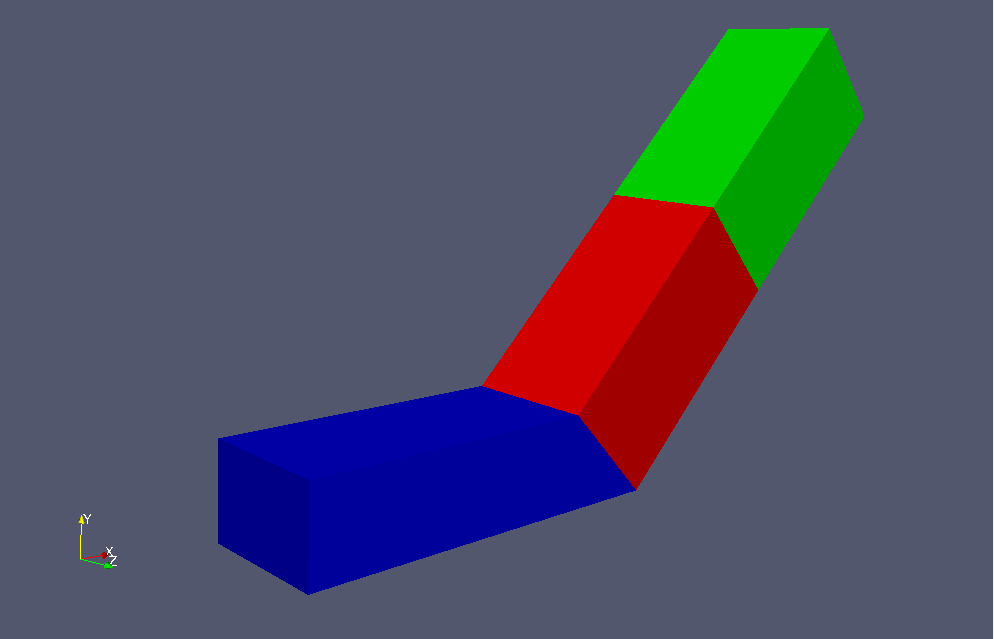Model geometry For porous media, a generator term S (pressure drop) according tois added to the Navier-Stokes equations. where ρref is the reference density and α and β are constant coefficients. The parameters that determine the properties of the porous media, the direction of the properties, and the region in which the porous media, are specified in the file constant/fvOptions as follows. porosity { type explicitPorositySource; active yes; explicitPorositySourceCoeffs { selectionMode cellZone; cellZone porosity; type fixedCoeff; active yes; cellZone porosity; fixedCoeffCoeffs { alpha (500 -1000 -1000); beta (0 0 0); rhoRef 1; coordinateSystem { type cartesian; origin (0 0 0); coordinateRotation { type axesRotation; e1 (0.70710678 0.70710678 0); e2 (0 0 1); } } } } }  The standard k-ε model is used for the turbulence model. The meshes are as follows, and the number of mesh is 22000.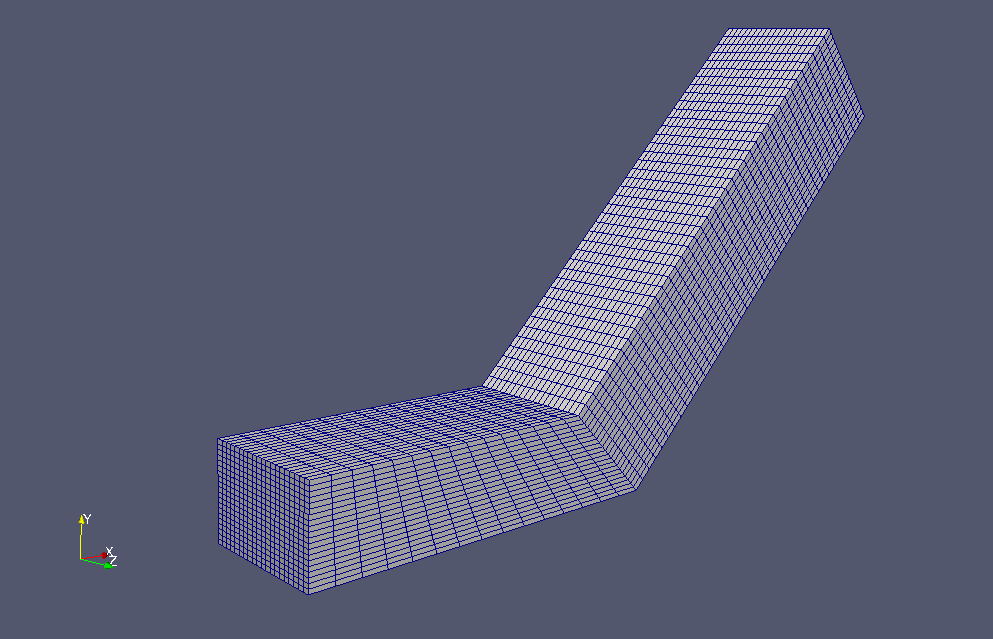Meshes If you want to visualize turbulent energy and turbulent dissipation rate, check "k" and "epsilon" in the "Properties" tab in ParaView.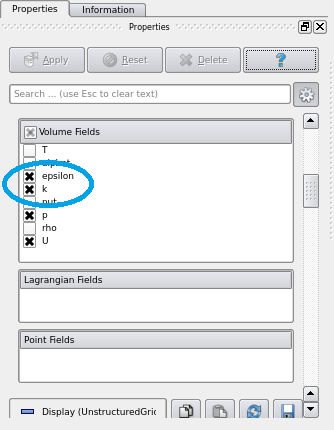Check "k" and "epsilon" in "Properties" tab The calculation result is as follows.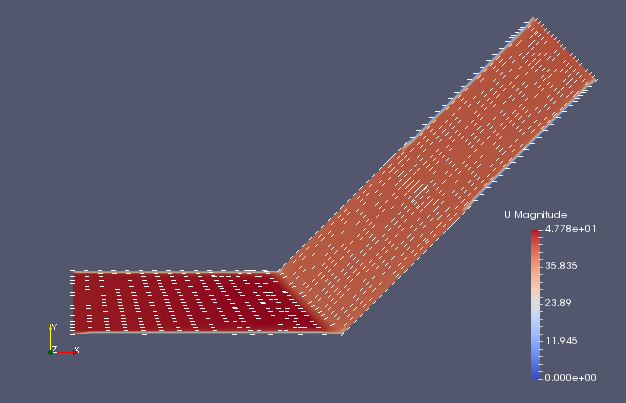Flow velocity (U)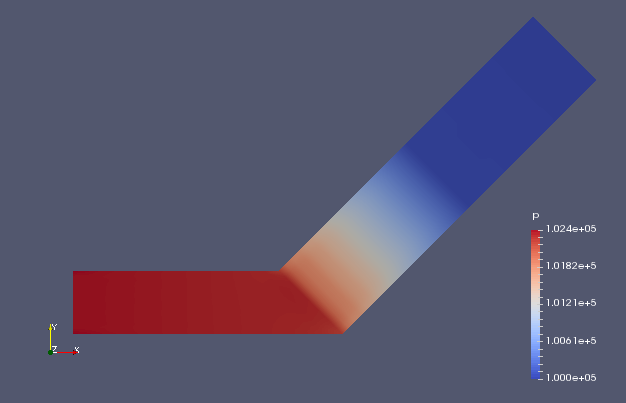Pressure (p)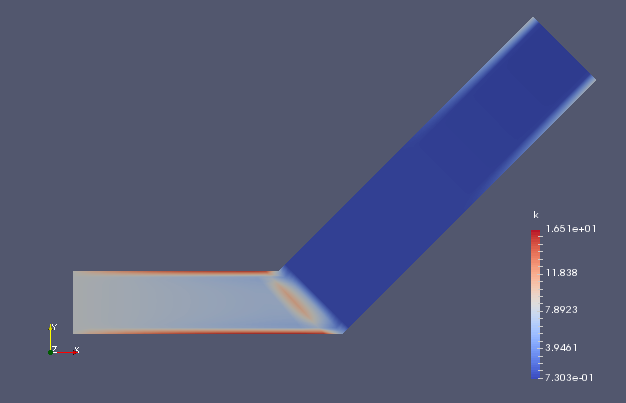Turbulent energy (k)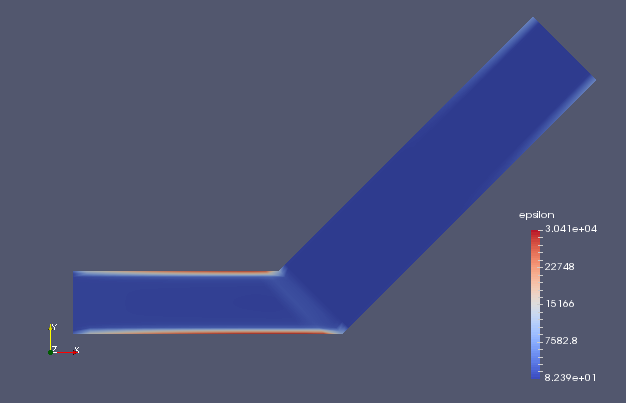Turbulent dissipation rate (epsilon) We can see that the flow velocity and pressure are greatly reduced in the porous media part. ## Commands cp -r$FOAM_TUTORIALS/compressible/rhoSimpleFoam/angledDuctExplicitFixedCoeff angledDuctExplicitFixedCoeff
cd angledDuctExplicitFixedCoeff

m4 system/blockMeshDict.m4 > system/blockMeshDict
blockMesh
rhoSimpleFoam

paraFoam

## Calculation time

24.97 seconds *Single, Inter(R) Core(TM) i7-8700 CPU @ 3.20GHz 3.19GHz